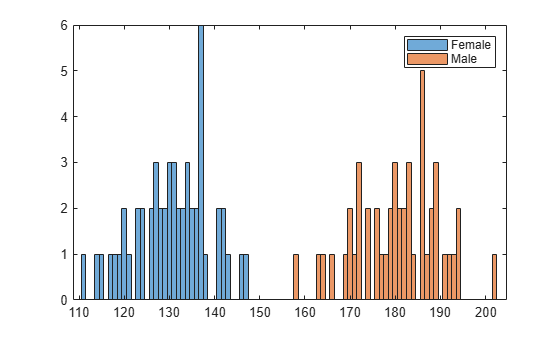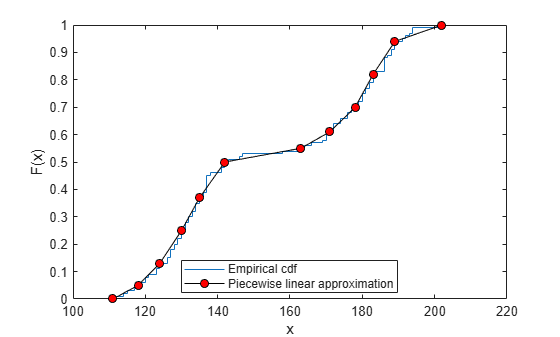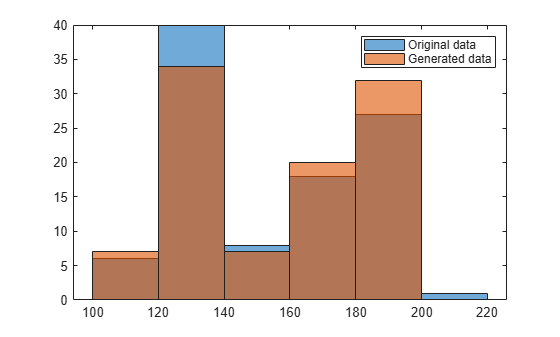Documentation

PiecewiseLinearDistribution

Piecewise linear probability distribution object

Description

A PiecewiseLinearDistribution object consists of a model description for a piecewise linear probability distribution.

The piecewise linear distribution is a nonparametric probability distribution created using a piecewise linear representation of the cumulative distribution function (cdf). The options specified for the piecewise linear distribution specify the form of the cdf. The probability density function (pdf) is a step function.

The piecewise linear distribution uses the following parameters.

ParameterDescription
xVector of x values at which the cdf changes slope
FxVector of cdf values that correspond to each value in x

Creation

Create a PiecewiseLinearDistribution probability distribution with specified parameter values object using makedist.

Properties

expand all

Distribution Parameters

Data values at which the cumulative distribution function (cdf) changes slope, specified as a vector of scalar values.

Data Types: single | double

cdf value at each value in x, specified as a vector of scalar values.

Data Types: single | double

Distribution Characteristics

This property is read-only.

Logical flag for truncated distribution, specified as a logical value. If IsTruncated equals 0, the distribution is not truncated. If IsTruncated equals 1, the distribution is truncated.

Data Types: logical

This property is read-only.

Number of parameters for the probability distribution, specified as a positive integer value.

Data Types: double

This property is read-only.

Distribution parameter values, specified as a vector.

Data Types: single | double

This property is read-only.

Truncation interval for the probability distribution, specified as a vector containing the lower and upper truncation boundaries.

Data Types: single | double

Other Object Properties

This property is read-only.

Probability distribution name, specified as a character vector.

Data Types: char

This property is read-only.

Distribution parameter descriptions, specified as a cell array of character vectors. Each cell contains a short description of one distribution parameter.

Data Types: char

This property is read-only.

Distribution parameter names, specified as a cell array of character vectors.

Data Types: char

Object Functions

 cdf Cumulative distribution function icdf Inverse cumulative distribution function iqr Interquartile range mean Mean of probability distribution median Median of probability distribution pdf Probability density function random Random numbers std Standard deviation of probability distribution truncate Truncate probability distribution object var Variance of probability distribution

Examples

collapse all

Create a piecewise linear distribution object using the default parameter values.

pd = makedist('PiecewiseLinear')
pd =
PiecewiseLinearDistribution

F(0) = 0
F(1) = 1

Load the sample data. Visualize the patient weight data using a histogram.

histogram(hospital.Weight)The histogram shows that the data has two modes, one for female patients and one for male patients.

Compute the empirical cumulative distribution function (ecdf) for the data.

[f,x] = ecdf(hospital.Weight);

Construct a piecewise linear approximation to the ecdf and plot both functions.

f = f(1:5:end); % keep a less dense grid of points
x = x(1:5:end);

figure;
ecdf(hospital.Weight)
hold on
plot(x,f,'ro','MarkerFace','r') % overlay grid
plot(x,f,'k') % show interpolationCreate a piecewise linear probability distribution object using the piecewise approximation of the ecdf.

pd = makedist('PiecewiseLinear','x',x,'Fx',f)
pd =
PiecewiseLinearDistribution

F(111) = 0
F(118) = 0.05
F(124) = 0.13
F(130) = 0.25
F(135) = 0.37
F(142) = 0.5
F(163) = 0.55
F(171) = 0.61
F(178) = 0.7
F(183) = 0.82
F(189) = 0.94
F(202) = 1

Generate 100 random numbers from the distribution.

rw = random(pd,100,1);

Plot the random numbers to visually compare their distribution to the original data.

figure;
histogram(rw)The random numbers generated from the piecewise linear distribution have the same bimodal distribution as the original data.